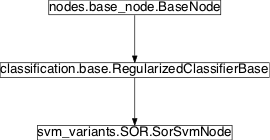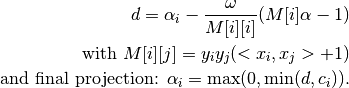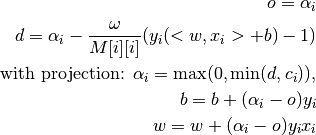# SOR¶

## Module: missions.nodes.classification.svm_variants.SOR¶

SVM variants using the SOR or dual gradient descent algorithm

All these variants have their offset in the target function. SOR is used as abbreviation for Successive Overrelaxation.

Inheritance diagram for pySPACE.missions.nodes.classification.svm_variants.SOR:## SorSvmNode¶

class pySPACE.missions.nodes.classification.svm_variants.SOR.SorSvmNode(random=False, omega=1.0, max_iterations=inf, version='samples', reduce_non_zeros=True, calc_looCV=False, squared_loss=False, offset_factor=1, **kwargs)[source]

Classify with 2-norm SVM relaxation using the SOR algorithm

This node extends the algorithm with some variants. SOR means successive overrelaxation. The offset b becomes part of the target function, which simplifies the optimization algorithm and allows for some dual gradient descent.

For further details, have a look at the given references and the reduced_descent which is an elemental processing step.

References

main source: M&M (matrix version)
author Mangasarian, O. L. and Musicant, David R.
title Successive Overrelaxation for Support Vector Machines
journal IEEE Transactions on Neural Networks
year 1998
volume 10
pages 1032–1037
minor source: Numerical Recipes (randomization)
author Press, William H. and Teukolsky, Saul A. and Vetterling, William T. and Flannery, Brian P.
title Numerical Recipes 3rd Edition: The Art of Scientific Computing
year 2007
isbn 0521880688, 9780521880688
edition 3
publisher Cambridge University Press
minor source: sample version
author Hsieh, Cho-Jui and Chang, Kai-Wei and Lin, Chih-Jen and Keerthi, S. Sathiya and Sundararajan, S.
title A dual coordinate descent method for large-scale linear SVM <http://doi.acm.org/10.1145/1390156.1390208>_
booktitle Proceedings of the 25th international conference on Machine learning
series ICML ‘08
year 2008
isbn 978-1-60558-205-4
location Helsinki, Finland
pages 408–415
numpages 8
doi 10.1145/1390156.1390208
acmid 1390208
publisher ACM

Parameters

Most parameters are already included into the RegularizedClassifierBase.

random:

Numerical recipes suggests to randomize the order of alpha. M&M suggest to sort the alpha by their magnitude.

(optional, default: False)

omega:

Descent factor of optimization algorithm. Should be between 0 and 2! Numerical recipes uses 1.3 and M&M choose 1.0.

(optional, default: 1.0)

version:

Using the matrix with the scalar products or using only the samples and track changes in w and b for fast calculations. Both versions give totally the same result but they are available for comparison. Samples is mostly a bit faster. For kernel usage only matrix is possible.

(optional, default: “samples”)

reduce_non_zeros:

In the inner loops, indices are rejected, if they loose there support.

(optional, default: True)

calc_looCV:

Calculate the leave-one-out metrics on the training data

(optional, default: False)

offset_factor:

Reciprocal weight, for offset treatment in the model

0: Use no offset Normal affine approach from augmented feature vectors Only small punishment of offset, enabling larger offsets (danger of numerical instability)

If 0 is used, the offset b is set to zero, otherwise it is used via augmented feature vectors with different augmentation factors. The augmentation value corresponds to 1/offset_factor, where 1/0 corresponds to infinity.

(optional, default: 1)

squared_loss:

Use L2 loss (optional) instead of L1 loss (default).

(optional, default: False)

In the implementation we do not use the name alpha but dual_solution for the variables of the dual optimization problem, which is optimized with this algorithm.

As a stopping criterion we use the maximum change to be less than some tolerance.

Exemplary Call

-
node : SOR
parameters :
complexity : 1.0
weight : [1,3]
debug : True
store : True
class_labels : ['Standard', 'Target']

Input: Output: FeatureVector PredictionVector Mario Michael Krell (mario.krell@dfki.de) 2012/06/27 SorSvmNode SorSvm SOR FeatureVector

Class Components Summary

 __hyperparameters _complete_training([debug]) Train the SVM with the SOR algorithm on the collected training data _execute(x) Executes the classifier on the given data vector in the linear case _stop_training([debug]) Forward process to complete training cycle add_new_sample(data[, class_label, default]) Add a new sample to the training set. append_weights_and_class_factors(label) Mapping between labels and weights/class factors calculate_weigts_and_class_factors() Calculate weights in the loss term and map label to -1 and 1 incremental_training(data, class_label) Warm Start Implementation by Mario Michael Krell input_types iteration_loop(M[, reduced_indices]) The algorithm is calling the reduced_descent method in loops over alpha looCV() Calculate leave one out metrics project(value, index) Projection method of soft_relax reduce_dual_weight(index) Change weight at index to zero reduced_descent(current_dual, M, ...) Basic iteration step over a set of indices, possibly subset of all remove_no_border_points(retraining_required) Discard method to remove all samples from the training set that are not in the border of their class. remove_non_support_vectors() Remove all samples that are no support vectors. remove_samples(idxs) Remove the samples at the given indices from the training set. retrain_SVM() Retrain the svm with the current training set total_descent(current_dual, M[, reduced_indices]) Different sorting of indices and iteration over all indices update_classification_function(delta, index) update classification function parameter w and b visualize() Show the training samples, the support vectors if possible and the current decision function.
__init__(random=False, omega=1.0, max_iterations=inf, version='samples', reduce_non_zeros=True, calc_looCV=False, squared_loss=False, offset_factor=1, **kwargs)[source]
_execute(x)[source]

Executes the classifier on the given data vector in the linear case

prediction value = <w,data>+b

_stop_training(debug=False)[source]

Forward process to complete training cycle

_complete_training(debug=False)[source]

Train the SVM with the SOR algorithm on the collected training data

looCV()[source]

Calculate leave one out metrics

reduce_dual_weight(index)[source]

Change weight at index to zero

calculate_weigts_and_class_factors()[source]

Calculate weights in the loss term and map label to -1 and 1

append_weights_and_class_factors(label)[source]

Mapping between labels and weights/class factors

The values are added to the corresponding list.

iteration_loop(M, reduced_indices=[])[source]

The algorithm is calling the reduced_descent method in loops over alpha

In the first step it uses a complete loop over all components of alpha and in the second inner loop only the non zero alpha are observed till come convergence criterion is reached.

reduced_indices will be skipped in observation.

reduced_descent(current_dual, M, relevant_indices)[source]

Basic iteration step over a set of indices, possibly subset of all

The main principle is to make a descent step with just one index, while fixing the other dual_solutions.

The main formula comes from M&M:Here we use c for the weights for each sample in the loss term, which is normally complexity times corresponding class weight. y is used for the labels, which have to be 1 or -1.

In the sample version only the diagonal of M is used. The sum with the alpha is tracked by using the classification vector w and the offset b.update_classification_function(delta, index)[source]

update classification function parameter w and b

project(value, index)[source]

Projection method of soft_relax

total_descent(current_dual, M, reduced_indices=[])[source]

Different sorting of indices and iteration over all indices

remove_no_border_points(retraining_required)[source]

Discard method to remove all samples from the training set that are not in the border of their class.

The border is determined by a minimum distance from the center of the class and a maximum distance.

Parameters: retraining_required – flag if retraining is required (the new point is a potential sv or a removed one was a sv)
add_new_sample(data, class_label=None, default=False)[source]

Add a new sample to the training set.

Parameters: data (list of float) – A new sample for the training set. class_label (str) – The label of the new sample. default – Specifies if the sample is added to the current training set or to a future training set default – bool
remove_samples(idxs)[source]

Remove the samples at the given indices from the training set.

Param: idxs: Indices of the samples to remove. idxs: list of int bool - True if a support vector was removed.
remove_non_support_vectors()[source]

Remove all samples that are no support vectors.

incremental_training(data, class_label)[source]

Warm Start Implementation by Mario Michael Krell

The saved status of the algorithm, including the Matrix M, is used as a starting point for the iteration. Only the problem has to be lifted up one dimension.

__hyperparameters = set([ChoiceParameter<kernel_type>, BooleanParameter<squared_loss>, NormalParameter<ratio>, NoOptimizationParameter<kwargs_warning>, NoOptimizationParameter<dtype>, NoOptimizationParameter<output_dim>, NoOptimizationParameter<use_list>, LogNormalParameter<epsilon>, BooleanParameter<regression>, NoOptimizationParameter<retrain>, LogUniformParameter<complexity>, ChoiceParameter<version>, NoOptimizationParameter<store>, NoOptimizationParameter<input_dim>, QNormalParameter<offset>, NoOptimizationParameter<debug>, QUniformParameter<max_time>, LogNormalParameter<tolerance>, UniformParameter<nu>, NoOptimizationParameter<keep_vectors>])
input_types = ['FeatureVector']
retrain_SVM()[source]

Retrain the svm with the current training set

visualize`()[source]

Show the training samples, the support vectors if possible and the current decision function.